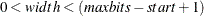### PackBits Call

`call PackBits` ( array, start, width, value ) ;

The PackBits call writes bits to a packed integer array. The inputs to the PackBits subroutine are as follows:

 array is an array to which the value is to be assigned. start is the starting position for the bit assignments. width is the number of bits to assign. value is the value to be assigned to the bits. For a single bit, this should be 0 or 1.

The PackBits subroutine facilitates the assignment of bit values into an integer array, effectively using the integer array as an array of bits. One common use for this routine is within a user genetic operator subroutine to pack bit values into an integer vector solution segment. The bit values assigned with the PackBits call can be retrieved with the UnpackBits function.

The start parameter is the lowest desired bit position in the bit vector, where the least significant bit of value is to be stored. The start parameter can range in value from 1 to maxbits, where maxbits is the product of 32 times the number of elements in the integer array.

The width parameter is the number of bits of value to be stored. It is bounded byand must also not exceed 32.

Bits not within the range defined by start and width are not changed. If the magnitude of value is too large to express in width bits, then the width least significant bits of value are transferred. The following program fragment, which might occur in a mutation subroutine, first reads a selected solution segment into `s` with the ReadMember call and then overwrites the first and second least significant bits of the solution with ones before writing it back to the current generation.

```   array s;